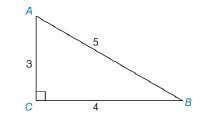Chapter 3.5, Problem 5E### Elementary Geometry for College St...

6th Edition
Daniel C. Alexander + 1 other
ISBN: 9781285195698

#### Solutions

Chapter
Section### Elementary Geometry for College St...

6th Edition
Daniel C. Alexander + 1 other
ISBN: 9781285195698
Textbook Problem
3 views

# In Exercise 1 to 10, classify each statement as true or false. m ∠ A + m ∠ B = m ∠ CTo determine

Whether the statement is true or false.

Explanation

Given:

The following figure shows the given diagram.

Given that,

mA+mB=mC

Approach:

The sum of interior angle of a triangle is 180°.

The equation for sum of interior angle of a triangle is as follows,

mA+mB+mC=180°

As shown in the figure that the C is 90°.

Hence,

### Still sussing out bartleby?

Check out a sample textbook solution.

See a sample solution

#### The Solution to Your Study Problems

Bartleby provides explanations to thousands of textbook problems written by our experts, many with advanced degrees!

Get Started

#### Simplify: 1339

Elementary Technical Mathematics

#### In Exercises 516, evaluate the given quantity. log4116

Finite Mathematics and Applied Calculus (MindTap Course List)

#### The area of the region at the right is:

Study Guide for Stewart's Multivariable Calculus, 8th